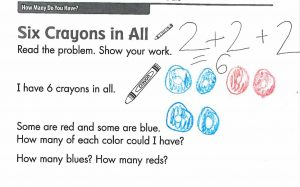Select Page

Classroom video is a powerful tool for studying and reflecting on mathematics teaching and learning.

Unlike in-the-moment interactions with students, watching video enables us to slow down and more closely examine student-to-student exchanges. This affords us a unique opportunity to learn about students’ mathematical thinking.

Several months ago, I began watching and discussing video footage of elementary mathematics classrooms with a group of colleagues. We were using the footage to study student engagement in the Standards for Mathematical Practice.

The task of watching video and identifying where students were enacting the Practices had an interesting effect on me. I began to notice students’ mathematical thinking in new ways.

For example, consider this video of two kindergarten students as they solve a story problem about 6 crayons, and use representations and notation to represent their solution.

Take a moment to think about what you noticed.

The first time I watched this clip, I noticed how these students persevered as they worked to record and then check their solution, all important aspects of Math Practice 1: make sense of problems and persevere in solving them. Moreover, these students engaged in this work with confidence, independence, and an expectation that they would be able to make sense of the problem together—aspects that made the clip compelling to watch.

As I watched it again, I began to see Math Practice 2: reason abstractly and quantitatively. This practice involves connecting symbols to the contexts they represent and moving back and forth between them. These kindergarteners worked hard to connect the equation 2+2+2=6 to their drawing of 2 groups of 2 blue crayons, and 1 group of 2 red crayons.A recreation of the students’ work

I watched a third time, and this time what stood out was Math Practice 4: model with mathematics. Both the drawing and the equation serve as mathematical models, and the students investigated the relationship between the mathematical elements depicted in each.

As I thought about which Practices the students were enacting, my appreciation for the complexity of the problem grew. For me, the clip illustrated that developing an understanding of mathematical notation is not merely about memorizing that 2 + 2 + 2 results in 6, but involves relating the symbolic notation to a context (in this case, 3 groups of 2 crayons).

Using the Practices as a lens through which to study students’ thinking highlighted the importance of making sense of the mathematics inherent in the task, for myself. Being able to pause and re-watch as I considered students’ approaches to the problem enabled me to notice more. If we value and are committed to deepening students’ mathematical ideas, learning how to notice children’s mathematical thinking is a critical part of our professional learning. Video is one tool that can be used outside of the classroom that I have found particularly useful in this work.

Tag(s): Kindergarten | notation | The Math Practices | video |